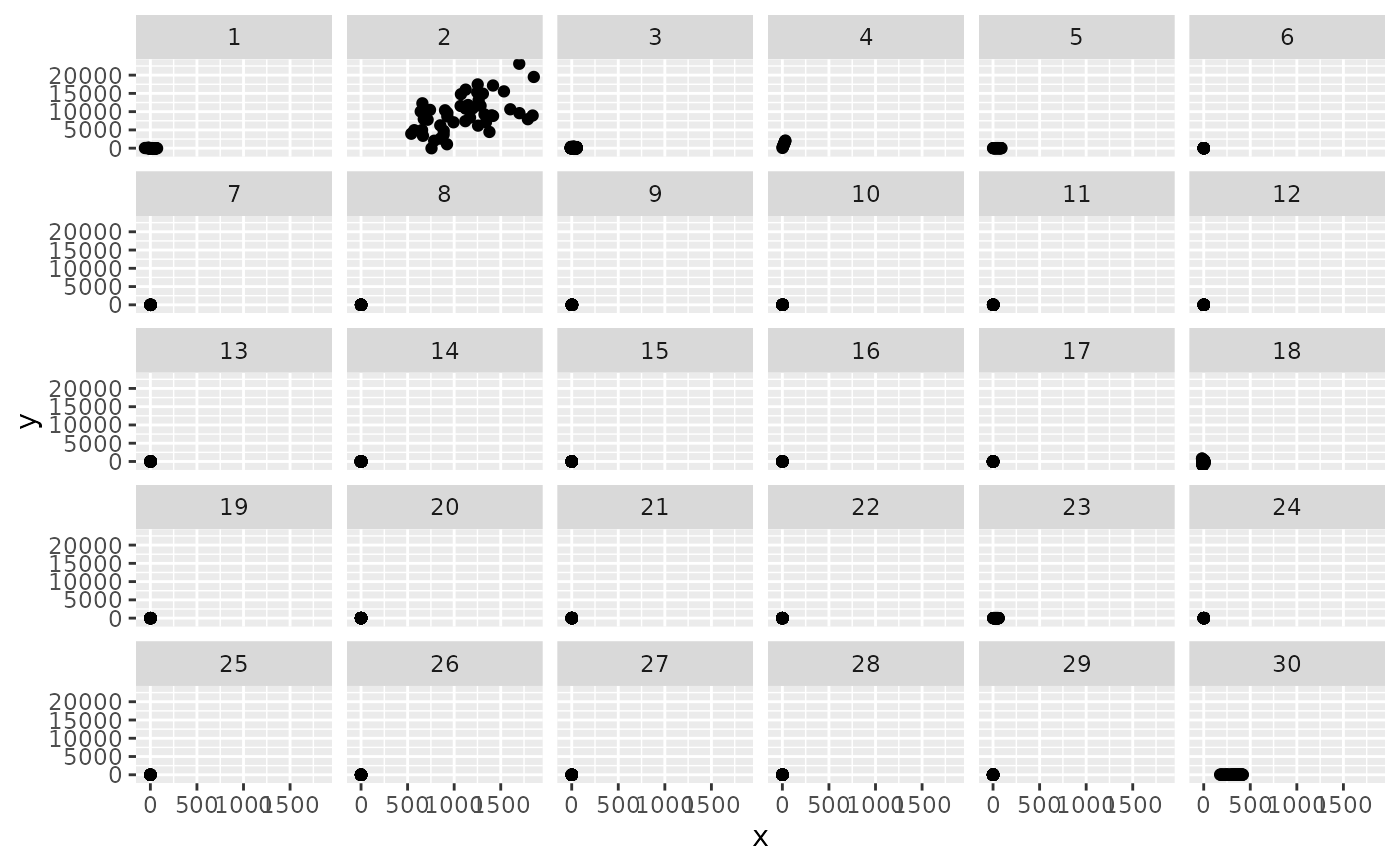Fake data.

## Usage

simulated_scatter

## Format

A data frame with 500 observations on the following 3 variables.

group

Group, representing data for a specific plot.

x

x-value.

y

y-value.

## Examples


library(ggplot2)

ggplot(simulated_scatter, aes(x = x, y = y)) +
geom_point() +
facet_wrap(~group)